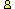CTK Exchange CTK Wiki Math Front Page Movie shortcuts Personal info Awards Terms of use Privacy Policy Cut The Knot! MSET99 Talk Games & Puzzles Arithmetic/Algebra Geometry Probability Eye Opener Analog Gadgets Inventor's Paradox Did you know?... Proofs Math as Language Things Impossible My Logo Math Poll Other Math sit's Guest book News sit's Recommend this siteCTK Exchange

 Subject: "Apollonian Circles Theorem" Previous Topic | Next Topic
 ConferencesThe CTK ExchangeThis and thatTopic #888Printer-friendly copyEmail this topic to a friend Reading Topic #888
mbsq
Member since Feb-2-09
Feb-02-09, 11:55 AM (EST)"Apollonian Circles Theorem"

 Hello,I was wondering if you could elaborate on the proof for the circles of Apollonius.(https://www.cut-the-knot.org/Curriculum/Geometry/LocusCircle.shtml)What argument did you have in mind behind the statement "For any P on the circle, the internal and external bisectors of angle APB pass through (the fixed points) M and N."?I came up with one but I am guessing yours is simpler. Here's mine:Take P on the circle, bisect the angle APB. If the bisector intersects the line AB at M' different from M, then assume WLOG M' is closer to B than is M. Then we get N' such that N'B/N'A = M'B/M'A and one can show that N' is closer to B than N is. Running the construction again we get a new circle with diameter M'N' properly within circle MN, but having intersection P, which is impossible.Is there a simpler one reasoning from similar triangles and the like?Thanks,Monroe

alexbCharter Member
2460 posts
Mar-09-09, 08:59 AM (EST)1. "RE: Apollonian Circles Theorem"
In response to message #0

 The bisector of an inscribed angle APB passes through the midpoint of the arc AB that does not contain P.mpdlcguest
Nov-20-09, 06:19 PM (EST)

2. "RE: Apollonian Circles Theorem"
In response to message #1

 Yesterday browsing in this marvelous site accidentally I ended on this page and took a look on the proof posted, I always learn new or something interesting. I challenge myself to find other proof more synthetic, below is my proposed proof based on projective geometry without drawing a line or writing an equation just reaoning, I appreciate take a look and tell me if it is correct of it has a flaw.As the ratio r express the absolute value of ratio of the segments AP/BP, there are two point M and N on the straight line AB which satisfy the above condition. Indeed r and minus r are the barycentric coordinates of M and N with respect to to A and B on line AB or in other words the simple ratio ABM and ABN are equal an opposed in sign.Therefore the cross ratio < ABMN> is harmonic being M and N conjugated.So if we draw a pencil of rays from any arbitrary point in the plane to the points A,B,M, and N the harmonic ratio will be preserved, however since point P must be selected so ray PM be the bisector of angle APB, the ray PN must be the other exterior bisector of the angle APB. All the above means that the angle NPM must be always 90 degrees and since MN is constant P must belong to a circumference of diameter MN.alexbCharter Member
2460 posts
Nov-21-09, 12:57 PM (EST)3. "RE: Apollonian Circles Theorem"
In response to message #2

 >I appreciate >take a look and tell me if it is correct of it has a flaw. I do not see a flaw, no. I can't say whether it is simpler or more elementary. What you do is replace the ratio property of angle bisectors (two of them being used simultaneously) with a property of harmonic ratio which also tackles two ratios simultaneously. Although it all depends on a view point, I would say that your suggestions is as simple as the proof on thepage but less elementary.For what it is worth... However, bringing in the harmonic ratio may suggest a generalization to the locus of a fixed cross-ratio. So it is always beneficial to have a second view.>As the ratio r express the absolute value of ratio of the >segments AP/BP, there are two point M and N on the straight >line AB which satisfy the above condition. Indeed r and >minus r are the barycentric coordinates of M and N with >respect to to A and B on line AB or in other words the >simple ratio ABM and ABN are equal an opposed in sign. >Therefore the cross ratio < ABMN> is harmonic being M and N >conjugated. >>So if we draw a pencil of rays from any arbitrary point in >the plane to the points A,B,M, and N the harmonic ratio will >be preserved, however since point P must be selected so ray >PM be the bisector of angle APB, the ray PN must be the >other exterior bisector of the angle APB. All the above >means that the angle NPM must be always 90 degrees and since >MN is constant P must belong to a circumference of diameter >MN.

 Conferences | Forums | Topics | Previous Topic | Next Topic
 Select another forum or conference Lobby The CTK Exchange (Conference)   |--Early math (Public)   |--Middle school (Public)   |--High school (Public)   |--College math (Public)   |--This and that (Public)   |--Guest book (Protected)   |--Thoughts and Suggestions (Public) Educational Press (Conference)   |--No Child Left Behind (Public)   |--Math Wars (Public)   |--Mathematics and general education (Public)You may be curious to have a look at the old CTK Exchange archive.